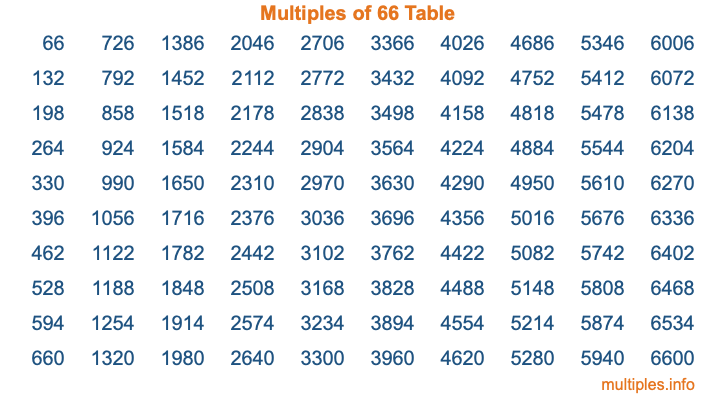Multiples of 66Welcome to the Multiples of 66 page. Here we will first teach you everything you will ever need to know about the multiples of 66, and then give you a study guide summary of everything we taught you to make sure you remember it all. Use this page to look up facts and learn information about the multiples of 66. This page will make you a multiples of sixty-six expert!

Definition of Multiples of 66
Multiples of 66 are all the numbers that when divided by 66 equal an integer. Each of the multiples of 66 are called a multiple. A multiple of 66 is created by multiplying 66 by an integer.

Therefore, to create a list of multiples of 66, you start with 1 multiplied by 66, then 2 multiplied by 66, then 3 multiplied by 66, and so on for as long as you want. Thus, the list of the first five multiples of 66 is 66, 132, 198, 264, and 330. To see a larger list of multiples of 66, see the printable image of Multiples of 66 further down on this page. We also have a category where you can choose any nth multiple of 66.

Multiples of 66 Checker
The Multiples of 66 Checker below checks to see if any number of your choice is a multiple of 66. In other words, it checks to see if there is any number (integer) that when multiplied by 66 will equal your number. To do that, we divide your number by 66. If the the quotient is an integer, then your number is a multiple of 66.

Is  a multiple of 66?

Least Common Multiple of 66 and ...
A Least Common Multiple (LCM) is the lowest multiple that two or more numbers have in common. This is also called the smallest common multiple or lowest common multiple and is useful to know when you are adding our subtracting fractions. Enter one or more numbers below (66 is already entered) to find the LCM.

Check out our LCM Calculator if you need more details about the Least Common Multiple or if you need the LCM for different numbers for adding and subtraction fractions.

nth Multiple of 66
As we stated above, 66 is the first multiple of 66, 132 is the second multiple of 66, 198 is the third multiple of 66, and so on. Enter a number below to find the nth multiple of 66.

th multiple of 66

Multiples of 66 vs Factors of 66
66 is a multiple of 66 and a factor of 66, but that is where the similarities end. All postive multiples of 66 are 66 or greater than 66. All positive factors of 66 are 66 or less than 66.

Below is the beginning list of multiples of 66 and the factors of 66 so you can compare:

Multiples of 66: 66, 132, 198, 264, 330, etc.

Factors of 66: 1, 2, 3, 6, 11, 22, 33, 66

As you can see, the multiples of 66 are all the numbers that you can divide by 66 to get a whole number. The factors of 66, on the other hand, are all the whole numbers that you can multiply by another whole number to get 66.

It's also interesting to note that if a number (x) is a factor of 66, then 66 will also be a multiple of that number (x).

Multiples of 66 vs Divisors of 66
The divisors of 66 are all the integers that 66 can be divided by evenly. Below is a list of the divisors of 66.

Divisors of 66: 1, 2, 3, 6, 11, 22, 33, 66

The interesting thing to note here is that if you take any multiple of 66 and divide it by a divisor of 66, you will see that the quotient is an integer.

Multiples of 66 Table
Below is an image of the first 100 multiples of 66 in a table. The table is in chronological order, column by column. The first column has the first ten multiples of 66, the second column has the next ten multiples of 66, and so on.The Multiples of 66 Table is also referred to as the 66 Times Table or Times Table of 66. You are welcome to print out our table for your studies.

Negative Multiples of 66
Although not often discussed or needed in math, it is worth mentioning that you can make a list of negative multiples of 66 by multiplying 66 by -1, then by -2, then by -3, and so on, to get the following list of negative multiples of 66:

-66, -132, -198, -264, -330, etc.

Multiples of 66 Summary
Below is a summary of important Multiples of 66 facts that we have discussed on this page. To retain the knowledge on this page, we recommend that you read through the summary and explain to yourself or a study partner why they hold true.

There are an infinite number of multiples of 66.

A multiple of 66 divided by 66 will equal a whole number.

66 divided by a factor of 66 equals a divisor of 66.

The nth multiple of 66 is n times 66.

The largest factor of 66 is equal to the first positive multiple of 66.

66 is a multiple of every factor of 66.

66 is a multiple of 66.

A multiple of 66 divided by a divisor of 66 equals an integer.

66 divided by a divisor of 66 equals a factor of 66.

Any integer times 66 will equal a multiple of 66.

Multiples of a Number
Here you can get the multiples of another number, all with the same attention to detail as we did for multiples of 66 on this page.

Multiples of
Multiples of 67
Did you find our page about multiples of sixty-six educational? Do you want more knowledge? Check out the multiples of the next number on our list!